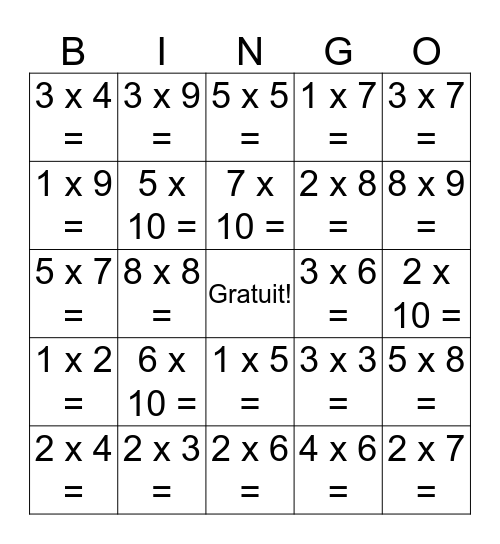# BingoThis bingo card has a free space and 54 words: 1 x 1 =, 1 x 2 =, 1 x 3 =, 1 x 4 =, 1 x 5 =, 1 x 6 =, 1 x 7 =, 1 x 8 =, 1 x 9 =, 1 x 10 =, 3 x 4 =, 2 x 2 =, 2 x 3 =, 2 x 4 =, 2 x 5 =, 2 x 6 =, 2 x 7 =, 2 x 8 =, 2 x 9 =, 2 x 10 =, 3 x 3 =, 3 x 5 =, 3 x 6 =, 3 x 7 =, 3 x 8 =, 3 x 9 =, 3 x 10 =, 4 x 4 =, 4 x 5 =, 4 x 6 =, 4 x 7 =, 4 x 8 =, 4 x 9 =, 4 x 10 =, 5 x 5 =, 5 x 6 =, 5 x 7 =, 5 x 8 =, 5 x 9 =, 5 x 10 =, 6 x 6 =, 6 x 7 =, 6 x 8 =, 6 x 9 =, 6 x 10 =, 7 x 7 =, 7 x 8 =, 7 x 9 =, 7 x 10 =, 8 x 8 =, 8 x 9 =, 8 x 10 =, 9 x 9 = and 9 x 10 =.

## Play Online